Apply receiver thermal noise to complex signal

•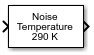Libraries:
Communications Toolbox / RF Impairments and Components

## Description

The Receiver Thermal Noise block applies receiver thermal noise to a complex signal. The block simulates the effects of thermal noise on a complex signal. The Specification method parameter enables specification of the thermal noise based on the noise temperature, noise figure, or noise factor.

## Examples

expand all

The `cm_receiver_thermal_noise_qam` model applies receiver thermal noise to a 16-QAM signal, and displays the signal in a constellation diagram. The model applies receiver thermal noise by specifying the noise figure in the Receiver Thermal Noise block.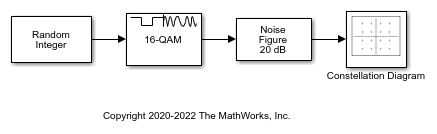Run the simulation at a sample rate of 1 kHz by setting the sample time to 1e-3 samples per second in the Random Integer Generator block. The Rectangular QAM Modulator Baseband block has the normalization method set to average power and the average power level set to 3e-13 watts (-92.5 dBm). Set the noise figure level in the Receiver Thermal Noise block to 20 dB, and display the constellation diagram of the 16-QAM signal.Decreasing the noise figure will reduce the simulated receive noise level, and result in a tighter clustering of samples for the individual points in the constellation diagram. To demonstrate this, set the noise figure level to 10 dB and show the constellation diagram of the 16-QAM signal.This example applies RF impairments to a signal modulated by the differential quadrature phase shift keying (DQPSK) method. To show the RF impairments, the example applies exaggerated levels that are not typical levels for modern radios.

In this example, the `slex_rcvrimpairments_dqpsk` model DQPSK-modulates a random signal and applies various RF impairments to the signal. The model uses impairment blocks from the RF Impairments library. The `InitFun` callback function initializes simulation variables. For more information, see Model Callbacks (Simulink).

After the impairment blocks, the signal forks into two paths. One path applies DC blocking, automatic gain control (AGC), and I/Q imbalance compensation to the signal before demodulation. The signal on the correction path is adjusted by the DC Blocker, AGC, and I/Q Imbalance Compensator blocks. Because the signal is DQPSK modulated, no carrier synchronization is required. The second path goes directly to demodulation. After demodulation, an error rate calculation is performed on both signals. The model includes Constellation Diagram blocks after modulation, before correction, and after correction so that you can analyze the constellation.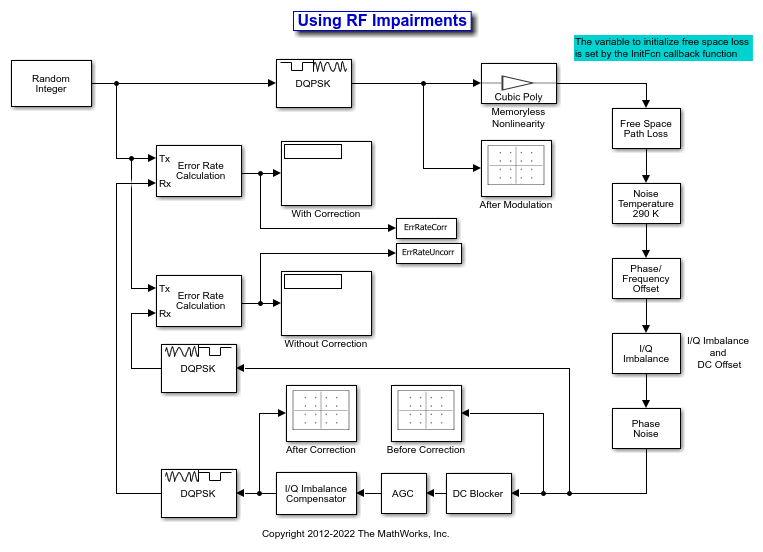When the model runs, constellation diagrams plot the signal at these stages in the simulation:

• The `After Modulation` constellation diagram shows the reference DQPSK-modulated signal constellation.

• The `Before Correction` constellation diagram shows the attenuated and distorted signal constellation.

• The `After Correction` constellation diagram shows the signal has been amplified and improved after the correction blocks.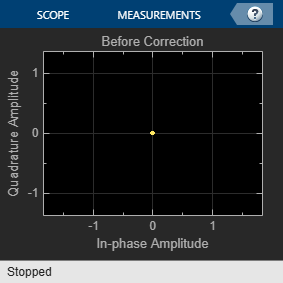The error rate for the demodulated signal without AGC is primarily caused by free space path loss and I/Q imbalance. The QPSK modulation minimizes the effects of the other impairments.

```Error rate for corrected signal: 0.000 Error rate for uncorrected signal: 0.042 ```

To explore the model try:

• Adjusting RF impairment settings, rerun the model, and notice the changes to the constellation diagrams and error rates.

• Modifying the model to add an equalizer stage before the demodulation. Equalization has inherent ability to reduce some of the distortion caused by impairments. For more information, see Equalization.

Apply thermal noise to a multichannel signal and compute the variance of each channel. Confirm the Receiver Thermal Noise block applies equivalent thermal noise to the individual channels by comparing the variances are approximately equal for each channel. The Receiver Thermal Noise block applies to confirm has the same noise floor by computing the variance of each channel.

The `multichan_thermal_noise.slx` model creates a multichannel signal with equal power per channel, and then applies receiver thermal noise to the multichannel signal.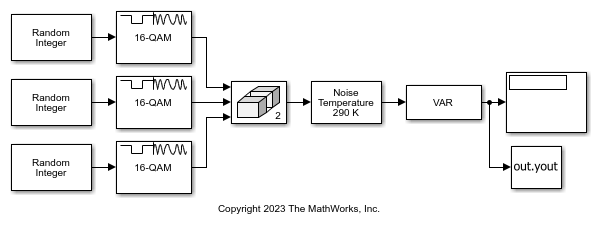Run the model to output the computed variance for each channel.

``` Ch1 Ch2 Ch3 Variance: 10.347 10.015 9.905 ```

## Ports

### Input

expand all

Baseband signal, specified as a complex-valued scalar, NS-element column vector, or NS-by-NC matrix. NS is the number of samples and NC is the number of channels.

Data Types: `double` | `single`
Complex Number Support: Yes

### Output

expand all

Output signal, returned as a scalar, column vector, or matrix of complex values with the same length and data type as the input signal.

## Parameters

expand all

To edit block parameters interactively, use the Property Inspector. From the Simulink® Toolstrip, on the Simulation tab, in the Prepare gallery, select .

Thermal noise specification method, specified as one of these options.

• `Noise temperature` specifies the noise in kelvins.

• `Noise figure` specifies the added receiver noise in dB relative to an input noise temperature of 290 K. The noise figure is the decibel equivalent of the noise factor.

• `Noise factor` specifies the added receiver noise relative to an input noise temperature of 290 K. The noise factor is the linear equivalent of the noise figure.

Noise temperature in kelvins, specified as a scalar.

Tunable: Yes

#### Dependencies

This parameter applies when you set Specification method to ```Noise temperature```.

Data Types: `double` | `single`

Receiver noise figure in dB relative to a noise temperature of 290 K, specified as a scalar.

Note

This parameter specifies the noise contribution of the receiver circuitry only. To add the effects of antenna noise, select Add 290K antenna noise.

Tunable: Yes

#### Dependencies

This parameter applies when you set Specification method to ```Noise figure```.

Data Types: `double` | `single`

Receiver noise factor relative to a noise temperature of 290 K, specified as a scalar.

Note

This parameter specifies the noise contribution of the receiver circuitry only. To add the effects of antenna noise, select Add 290K antenna noise.

Tunable: Yes

#### Dependencies

This parameter applies when you set Specification method to ```Noise factor```.

Data Types: `double` | `single`

Select this parameter to add 290 K antenna noise to the signal.

#### Dependencies

This parameter applies when you set Specification method to ```Noise factor``` or ```Noise figure```.

Reference load value in ohms, specified as a scalar. This value is used to compute the voltage levels based on the signal and noise power levels.

Tunable: Yes

Data Types: `double` | `single`

Source for random number stream, specified as ```Global stream``` or ```mt19937ar with seed```.

#### Limitations

To use this block in a For Each Subsystem (Simulink) you must set this parameter to `Global Stream`. This ensures that each run will generate independent noise samples.

Initial seed value for the random number generator, specified as a scalar.

#### Dependencies

This parameter applies when you set Random number source to ```mt19937ar with seed```.

Data Types: `double` | `single`

Type of simulation to run, specified as `Code generation` or `Interpreted execution`.

• `Code generation` — Simulate the model by using generated C code. The first time you run a simulation, Simulink generates C code for the block. The model reuses the C code for subsequent simulations unless the model changes. This option requires additional startup time, but the speed of the subsequent simulations is faster than with the `Interpreted execution` option.

• `Interpreted execution` — Simulate the model by using the MATLAB® interpreter. This option shortens startup time, but the speed of subsequent simulations is slower than with the ```Code generation``` option. In this mode, you can debug the source code of the block.

## Block Characteristics

 Data Types `double` | `single` Multidimensional Signals `no` Variable-Size Signals `yes`

## Algorithms

Wireless receiver performance is often expressed as a noise factor or figure. The noise factor, F, is defined as the ratio of the input signal-to-noise ratio, Si/Ni to the output signal-to-noise ratio, So/No, such that

`$F=\frac{{S}_{i}/{N}_{i}}{{S}_{o}/{N}_{o}}\text{\hspace{0.17em}}.$`

Given the receiver gain G and receiver noise power Nckt, the noise factor can be expressed as

`$\begin{array}{c}F=\frac{{S}_{i}/{N}_{i}}{G{S}_{i}/\left({N}_{ckt}+G{N}_{i}\right)}\\ =\frac{{N}_{ckt}+G{N}_{i}}{G{N}_{i}}\text{\hspace{0.17em}}.\end{array}$`

The IEEE® defines the noise factor assuming that noise temperature at the input is T0, where T0 = 290 K. The noise factor is then

`$\begin{array}{c}F=\frac{{N}_{ckt}+G{N}_{i}}{G{N}_{i}}\\ =\frac{GkB{T}_{ckt}+GkB{T}_{0}}{GkB{T}_{0}}\\ =\frac{{T}_{ckt}+{T}_{0}}{{T}_{0}}\text{\hspace{0.17em}}.\end{array}$`

k is Boltzmann's constant. B is the signal bandwidth. Tckt is the equivalent input noise temperature of the receiver and is expressed as

`${T}_{ckt}={T}_{0}\left(F-1\right)\text{\hspace{0.17em}}.$`

The overall noise temperature of an antenna and receiver Tsys is

`${T}_{sys}={T}_{ant}+{T}_{ckt}\text{\hspace{0.17em}},$`

where Tant is the antenna noise temperature.

The noise figure NF is the dB equivalent of the noise factor and can be expressed as

`$NF=10{\mathrm{log}}_{10}\left(F\right)\text{\hspace{0.17em}}.$`

The noise power can be expressed as

`$N=kTB={V}^{2}/R,$`

where V is the noise voltage expressed as

`${V}^{2}=kTBR,$`

and R is the reference load.

## Version History

Introduced before R2006a

expand all